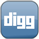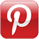### Ternary OperatorsJavaScript supports a ternary operator-an operator that exists as a shortcut for if/else.

The ternary operator returns one of two possible expressions depending on a conditional check.
``` condition ? expression1 : expression2 ```
So let's look at a typical example done with an if/else block.
``` if (totalPrice > 100) {     discount=.05; } else {     discount=0; } ```
Now, let's look at the same code using a ternary operator.
``` discount = totalPrice > 100 ? .05 : 0; ```